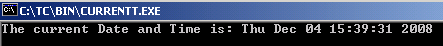Tutorials

# C Current Time

The header file provides all date and time functions. In the given example, the time() function determines the current time in seconds and assigns it to time_t type variable t.

The header file provides all date and time functions. In the given example, the time() function determines the current time in seconds and assigns it to time_t type variable t.

# C Current Time

In this section, you will learn how to get the current time in C.

The header file <time.h> provides all date and time functions. In the given example, the time() function determines the current time in seconds and assigns it to time_t type variable t. The ctime() function converts the number of seconds to the current date and time. The printf() function then prints the current date and time.

Here is the code:

CURRENTT.C

 ``` #include  #include  #include  void main() {   time_t t;   time(&t);   printf("The current Date and Time is: %s\n", ctime(&t));   getch(); }```

Output will be displayed as:

CURRENTT.EXE